# To graph : The quadratic equation y = 100 − x 2 in a graphing window.### Precalculus: Mathematics for Calcu...

6th Edition
Stewart + 5 others
Publisher: Cengage Learning
ISBN: 9780840068071### Precalculus: Mathematics for Calcu...

6th Edition
Stewart + 5 others
Publisher: Cengage Learning
ISBN: 9780840068071

#### Solutions

Chapter 1.9, Problem 7E
To determine

## To graph: The quadratic equation y=100−x2 in a graphing window.

Expert Solution

### Explanation of Solution

Given information:

Graph:

The graph of the quadratic equation y=100x2 can be sketched in the cartesian plane,

Consider the quadratic equation, y=100x2 .

Now put the values of x in the equation to find the values of y or to find the real roots of y with the help of x .

Solve the equation y=100x2 with the quadratic formula:

b±b24ac2a

Now solve the equation y=100x2 by quadratic formula:

Find the value of a,b,c :

The value of a,b,c are , a is the coefficient of x2 , b is the coefficient of x , c is constant.

Thus, the value of:

a=1b=0c=100

Substitute the values of a,b,c in quadratic formula:

b±b24ac2a

b±b24ac2a=0±4×1×1002×1=±4002=±202

Either the value ±202 is positive or negative:

When the value ±202 is positive, then the real root is 10 ,

When the value ±202 is negative, then the real root is 10 ,

So x=10 or x=10 ,

Here observed the equation of parabola, the coefficient of x2 is negative, so the graph is opened downward.

The roots and the shape of parabola is cleared.

The graph of the equation y=100x2 .

Substitute x=0 , in the equation y=100x2 ,

y=100x2y=100(0)2y=100

Substitute x=1 , in the equation y=100x2 ,

y=100x2y=100(1)2y=1001y=99

Substitute x=2 , in the equation y=100x2 ,

y=100x2y=100(2)2y=1004y=96

Observe that as the value of x increases there is the decrement in value of y .

Steps to plot the graph of the equation y=100x2 with the help of graphing utility are as follows:

Step 1: Press MODE key.

Step 2: Use the down arrow key to reach FUNC option.

Step 3: Press ENTER key.

Step 4: Press Y= key.

Step 5: Enter the function y=100x2 .

Step 6: Press WINDOW key. Change the settings to

Xmin=100Xmax=100Ymin=100Ymax=150

For better view of graph.

Step 8: Press GRAPH key.

The result obtained on the screen is provided below,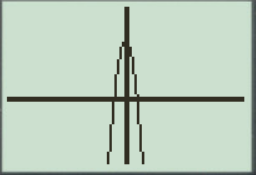Interpretation:

The equation of the function y=100x2 represents a parabola.

The parabola opens downward.

The x intercepts are the points on xaxis where the graph of the equation touches xaxis .

Therefore, in the equation, y=100x2 x intercepts are [10,10] .

Therefore, the equation y=100x2 is symmetric about the y axis Initially the graph of the equation y=100x2 decreases when increase the value of x ,the graph is a parabola shaped graph.

a.

When the x -axis ranges from [4,4] and y -axis ranges from [4,4] their graph obtained in the screen is provided below.

Steps to plot the graph of the equation y=100x2 with the help of graphing utility are as follows:

Step 1: Press MODE key.

Step 2: Use the down arrow key to reach FUNC option.

Step 3: Press ENTER key.

Step 4: Press Y= key.

Step 5: Enter the function y=100x2 .

Step 6: Press WINDOW key. Change the settings to

Xmin=4Xmax=4Ymin=4Ymax=4

For better view of graph.

Step 8: Press GRAPH key.

The result obtained on the screen is provided below,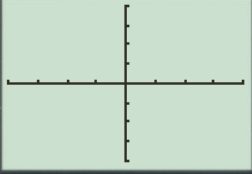This window is not perfect viewing window.

b.

When the x -axis ranges from [10,10] and y -axis ranges from [10,10] their graph obtained in the screen is provided below.

Steps to plot the graph of the equation y=100x2 with the help of graphing utility are as follows:

Step 1: Press MODE key.

Step 2: Use the down arrow key to reach FUNC option.

Step 3: Press ENTER key.

Step 4: Press Y= key.

Step 5: Enter the function y=100x2 .

Step 6: Press WINDOW key. Change the settings to

Xmin=10Xmax=10Ymin=10Ymax=10

For better view of graph.

Step 8: Press GRAPH key.

The result obtained on the screen is provided below,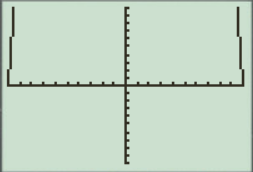This window is not a perfect viewing window.

c.

When the x -axis ranges from [15,15] and y -axis ranges from [30,110] their graph obtained in the screen is provided below.

Steps to plot the graph of the equation y=100x2 with the help of graphing utility are as follows:

Step 1: Press MODE key.

Step 2: Use the down arrow key to reach FUNC option.

Step 3: Press ENTER key.

Step 4: Press Y= key.

Step 5: Enter the function y=100x2 .

Step 6: Press WINDOW key. Change the settings to

Xmin=15Xmax=15Ymin=30Ymax=110

For better view of graph.

Step 8: Press GRAPH key.

The result obtained on the screen is provided below,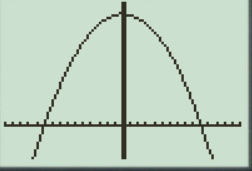This is the perfect viewing window.

d.

When the x -axis ranges from [4,4] and y -axis ranges from [30,110] their graph obtained in the screen is provided below.

Steps to plot the graph of the equation y=100x2 with the help of graphing utility are as follows:

Step 1: Press MODE key.

Step 2: Use the down arrow key to reach FUNC option.

Step 3: Press ENTER key.

Step 4: Press Y= key.

Step 5: Enter the function y=100x2 .

Step 6: Press WINDOW key. Change the settings to

Xmin=4Xmax=4Ymin=30Ymax=110

For better view of graph.

Step 8: Press GRAPH key.

The result obtained on the screen is provided below,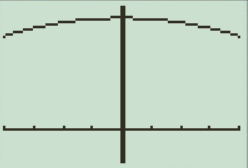This window has large view, in this the graph points are not clear.

So perfect viewing window of the graph y=100x2 is option ‘c’ [15,15]by[30,110] .

Thus, option ‘c’ [15,15]by[30,110] has a perfect viewing window of the equation y=100x2 .

### Have a homework question?

Subscribe to bartleby learn! Ask subject matter experts 30 homework questions each month. Plus, you’ll have access to millions of step-by-step textbook answers!